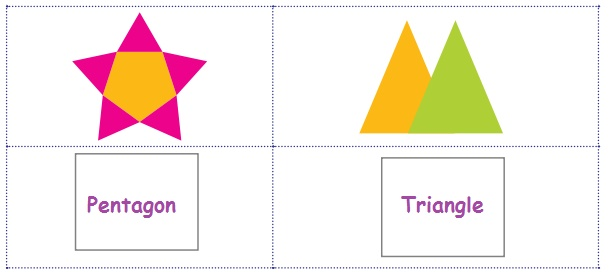Home | | Maths 4th Std | Exercise 1 (Properties of 2-D Shaped objects)

# Exercise 1 (Properties of 2-D Shaped objects)

4th Maths : Term 1 Unit 1 : Geometry : Book Back, Exercise, Example Numerical Question with Answers, Solution

Exercise 1

A. Write down the names of shape in the following pictures.B.

1. Write the number of squares and triangles in the given picture.2. Write the number of rectangles and triangles in the given picture.3. Identify the cut shapes and write the names in the boxes given below.Tags : Geometry | Term 1 Chapter 1 | 4th Maths , 4th Maths : Term 1 Unit 1 : Geometry
Study Material, Lecturing Notes, Assignment, Reference, Wiki description explanation, brief detail
4th Maths : Term 1 Unit 1 : Geometry : Exercise 1 (Properties of 2-D Shaped objects) | Geometry | Term 1 Chapter 1 | 4th Maths